# Semi Bluffing Examples

The Semi Bluff > Semi Bluffing EV Examples

Here are a two examples that highlight how it’s more profitable (+EV) to bluff with semi bluffs as opposed to “total bluffs”.

1. Cbetting the flop as a bluff.
2. Check-raising the turn as a bluff.

If you're not familiar with working out EV, you should check out the article on expected value first.

## 1) Cbetting the flop as a bluff.

We raise to 3bb from late position in a game of \$25NL. Everyone else folds except for the BB who calls.

Flop: K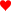T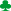4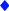Pot: \$1.60

Our opponent checks and the action is on us.

Let’s assume that we know for a fact that if we make a cbet of \$1.20, our opponent is going to fold their hand 40% of the time. When our opponent does call the other 60% of the time, they will have any pair, mid-pocket pair, OESD or better. So a fair reflection of their calling range looks like this:Our opponent's calling range against our cbet on a KT4 flop.

Note: I left out AK and AKs because we're assuming that they would 3bet those hands preflop.

To keep it simple, let’s also say that we know that our opponent is not going to check-raise us on the flop. In addition, if our cbet gets called we will both check the turn and river to see a showdown (unlikely, but the EV is the most important thing here).

Let’s look at the EV of bluffing with different types of hands in this situation.

1. 72o - virtually a total bluff.
2. A9o - a fairly weak semi bluff (just one over card to the board).
3. QJ- a strong semi bluff (open ended straight draw and backdoor flush draw).

### a) 72o - virtually a total bluff.

Our equity against our opponent’s calling range with 72o = 5%.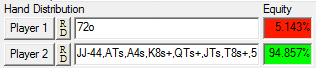Here are the different possible outcomes and the amount of money we expect to win/lose on average from each.

• FOLD EQUITY = Our opponent folds.
• We win \$1.60, 40% of the time.
• \$1.60 x 0.4 = \$0.64.
• POT EQUITY 1 = Our opponent calls and we improve to make the best hand.
• We win \$2.80 (\$1.60 + \$1.20) 5% of the time.
• \$2.80 x 0.05 = \$0.14
• POT EQUITY 2 = Our opponent calls and we don’t improve.
• We lose \$1.20 95% of the time.
• -\$1.20 x 0.95 = -\$1.14

The last two outcomes (the overall pot equity result) will happen 60% of the time. Therefore, we will need to multiply these two results by 60% (0.6) to account for the fact that they only happen a portion of the time.

• EV of bluffing with 72o.
• EV = Fold Equity + Pot Equity
• EV = (\$0.64) + { 0.6 x [ (\$0.14) - (\$1.14) ] }
• EV = (\$0.64) + { -\$0.60}
• EV = +\$0.04

### b) A9o - a fairly weak semi bluff (just one over card to the board).

Our equity against our opponent’s calling range with A9o = 18%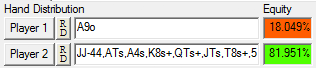• EV of cbet bluffing with A9o
• EV = Fold Equity + Pot Equity
• EV = (\$1.60 x 0.4) + { 0.6 x [ (\$2.80 x 0.18) + (-\$1.20 x 0.82) ] }
• EV = (\$0.64) + { 0.6 x [ (\$0.50) - (\$0.98) ] }
• EV = (\$0.64) + { -\$0.29 }
• EV = +\$0.35

### c) QJ- a strong semi bluff (open ended straight draw and backdoor flush draw).

Our equity against our opponent’s calling range with QJ= 45%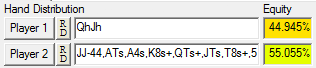• EV of cbet bluffing with QJ• EV = Fold Equity + Pot Equity
• EV = (\$1.60 x 0.4) + { 0.6 x [ (\$2.80 x 0.45) + (-\$1.20 x 0.55) ] }
• EV = (\$0.64) + { 0.6 x [ (\$1.12) - (\$0.66) ] }
• EV = (\$0.64) + {\$0.28}
• EV = +\$0.92

As you can see from this very basic cbetting example, the presence of equity can turn a borderline -EV play in to a highly +EV play. You will probably find the difference in EV for each of these cbet bluffs to be very surprising.

They’re all bluffs at the end of the day, but bluffing with equity is much better than bluffing without it.

If you're struggling with when and when not to cbet as a bluff, watch this c-betting strategy video by SplitSuit.

## 2) Check raising the turn as a bluff.

An opponent makes it 4bb from the button in \$100NL. We call in the BB and everyone else folds.

Flop: 7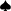8QPot: \$8.50

We check, our opponent bets \$5.50 in to the pot and we call.

Turn: 78QKPot: \$19.50

We check and our opponent bets \$13.

Pot: \$32.50

We only have \$50 left in our stack, so we decide to check-raise bluff all in. This means our opponent has to call \$37 to win a pot of \$82.5.

Pot: \$82.50

We expect our opponent to fold 35% of the time. However, the remaining 65% of the time our opponent will call with AQ, any K with a strong kicker and strong draws or better. Therefore, their range will look something like this: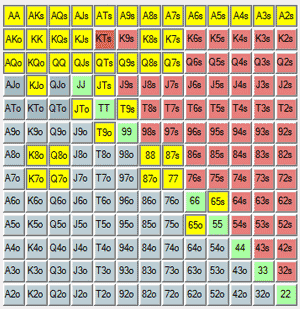Our opponent's calling range after we check/raise all in on the turn.

What does the EV look like for different bluffs and semi bluffs in this situation?

1. 32o - virtually a total bluff.
2. T9o - a decent semi bluff (middle straight draw).
3. JT- a strong semi bluff (nut straight draw + decent flush draw).

### a) 32o - virtually a total bluff.

Our equity against our opponent’s calling range with 32o = 7%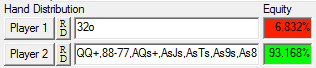• FOLD EQUITY = Our opponent folds.
• We win \$32.50 (this is what’s currently in the pot) 35% of the time.
• \$32.50 x 0.35 = \$11.38.
• POT EQUITY 1 = Our opponent calls and we improve to make the best hand.
• We win \$69.50 (\$32.50 + \$37) 7% of the time.
• \$69.50 x 0.07 = \$4.9
• POT EQUITY 2 = Our opponent calls and we don’t improve.
• We lose \$50 93% of the time.
• -\$50 x 0.93 = -\$46.5

Again, don't forget that the the amount we win and lose when called only happens a weighted portion of the time. Therefore, we have to multiply the Pot Equity result by the overall likelihood that it takes place, which is 65%.

• EV of cbet bluffing with 72o
• EV = Fold Equity + Pot Equity
• EV = (\$11.38 + { 0.65 x [ (\$4.9) - (\$46.5) ] }
• EV = (\$11.38 + {-\$27.04 }
• EV = -\$15.66

### b) T9o - a decent semi bluff (middle straight draw).

Our equity against our opponent’s calling range with T9o = 26%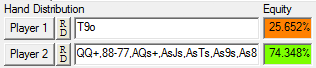• EV of cbet bluffing with T9o
• EV = Fold Equity + Pot Equity
• EV = (\$32.50 x 0.35) + { 0.65 x [ (\$69.50 x 0.26) + (-\$50 x 0.74) ] }
• EV = (\$11.38) + { 0.65 x [ (\$18.07) - (\$37) ] }
• EV = (\$11.38) + {-\$12.30}
• EV = -\$0.92

### c) JT- a strong semi bluff (nut straight draw + decent flush draw).

Our equity against our opponent’s calling range with JT= 43%• EV of cbet bluffing with JT• EV = Fold Equity + Pot Equity
• EV = (\$32.50 x 0.35) + { 0.65 x [ (\$69.50 x 0.43) + (-\$50 x 0.57) ] }
• EV = (\$11.38) + { 0.65 x [ (\$29.89) - (\$28.5) ] }
• EV = (\$11.38) + {\$0.90}
• EV = \$12.28

In this example, we need to have a good amount of equity in the hand for this bluff to be successful due to the fact that our opponent is calling quite frequently with a strong range. If our opponent was more likely to fold, semi-bluffing with the T9o could have become slightly +EV rather than -EV.

## Important notes.

1) When working out the final EV for semi bluffing, don't forget to multiply the combined EV from winning and losing the hand after being called by the percentage chance of being called in the first place. If you do not do this the results will be skewed.

2) The first cbetting example isn’t great because it assumes that you will see a river card for free and that you won’t bet if you hit your draw. However, it is useful to note that implied odds (winning more money when you hit) can help to turn slightly -EV plays in the +EV plays.

3) The less likely it is for your opponent to fold (the less fold equity you have), the more actual equity you need in the hand to make semi-bluffing profitable.

4) If you have no fold equity with a drawing hand in position, you’re better off checking to see a free card. There is no value is putting more money in to the pot if you have no fold equity and just a draw.

5) Don’t forget that reverse implied odds will have an effect on your equity in the hand if you get called. It’s not a great idea to semi-bluff with weak flushes for example because your opponent could be drawing to a bigger flush.

## Further reading on the EV of semi-bluffing.

Getting your head around the EV involved in semi-bluffs is tricky to say the least. If my particular teaching method hasn't worked for you, try having a read through these pages:

Go back to the awesome Texas Hold'em Strategy.

Can You Afford Not To Use
Poker Tracker 4?“I wouldn’t play another session of online poker without it”

“I play \$25NL, and in under 1 week PT4 had paid for itself”